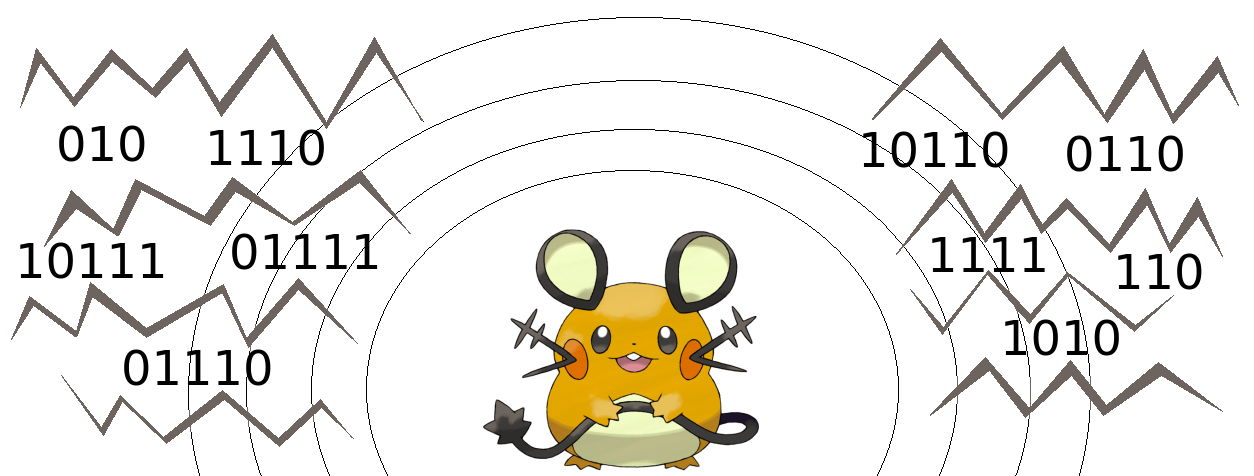시간 제한메모리 제한제출정답맞힌 사람정답 비율
5 초 512 MB27151164.706%

## 문제

Have you ever heard about the famous Dedenne antennas? They allow them to communicate with each other even when they’re miles and miles apart. However, they suspect that they don’t communicate in an optimal way. That’s why Dedenne came to you in order to optimize their language.

The language used by Dedenne consists of n words. In order to transmit a word, Dedenne must first use their memorized dictionary to convert the word into a non-empty binary string (a codeword) and then transmit the resulting string. The recipient will then receive the sequence and use the same dictionary to recover the word. In order to preserve the correctness of the transmission, no two codewords in the dictionary should be equal or even no string should be a prefix of any other string.

Due to the peculiar nature of Dedenne, the codewords aren’t allowed to have two consecutive 0 bits — when transmitted, they merge together into ∞ and hang up the transmitter indefinitely.

The dictionary is obviously quite painful to memorize. Formally speaking, let C(s) be the cost of any binary string s, equal to exactly $$\sum_{j=1}^{k}{\lfloor1 + \log_2{j}\rfloor}$$ if s is the prefix of exactly k codewords. Then, the cost to memorize the dictionary is equal to the sum of C(s) over all binary strings s which are the prefixes of at least one codeword.

For example, consider the dictionary consisting of 4 codewords: 0, 10, 110, 111. We can now see that the cost of memorizing the dictionary is equal to C(ε) + C(0) + C(1) + C(10) + C(11) + C(110) + C(111) = 8 + 1 + 5 + 1 + 3 + 1 + 1 = 20. Note that ε denotes an empty word.

Now you probably know what Dedenne want from you. What is the minimum possible cost of memorizing the dictionary?

## 입력

The first line contains a single integer t (1 ≤ t ≤ 50 000) — the number of independent testcases. Each of the following t lines describes a single testcase and contains one integer n (2 ≤ n ≤ 1015) — the number of words that Dedenne need to memorize.

## 출력

For each testcase, output a single integer — the minimum cost of a dictionary consisting of n words that can be achieved by Dedenne.

## 예제 입력 1

3
2
4
10


## 예제 출력 1

5
20
98


## 힌트

Here is a dictionary which might be used by Dedenne for n = 10 to minimize the cost of memorizing: